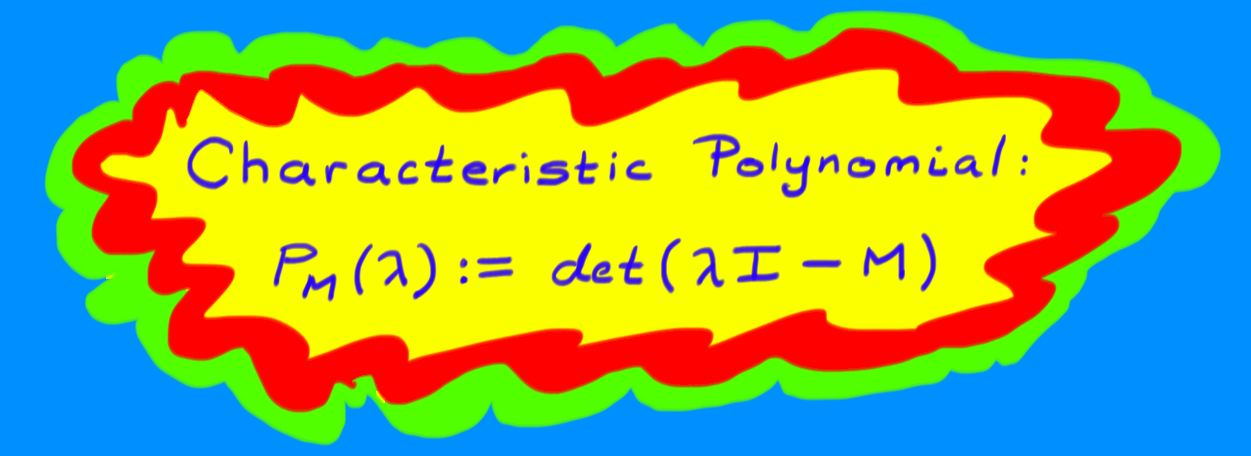$$\newcommand{\id}{\mathrm{id}}$$ $$\newcommand{\Span}{\mathrm{span}}$$ $$\newcommand{\kernel}{\mathrm{null}\,}$$ $$\newcommand{\range}{\mathrm{range}\,}$$ $$\newcommand{\RealPart}{\mathrm{Re}}$$ $$\newcommand{\ImaginaryPart}{\mathrm{Im}}$$ $$\newcommand{\Argument}{\mathrm{Arg}}$$ $$\newcommand{\norm}{\| #1 \|}$$ $$\newcommand{\inner}{\langle #1, #2 \rangle}$$ $$\newcommand{\Span}{\mathrm{span}}$$

# 12.2: The Eigenvalue-Eigenvector Equation

$$\newcommand{\vecs}{\overset { \rightharpoonup} {\mathbf{#1}} }$$ $$\newcommand{\vecd}{\overset{-\!-\!\rightharpoonup}{\vphantom{a}\smash {#1}}}$$$$\newcommand{\id}{\mathrm{id}}$$ $$\newcommand{\Span}{\mathrm{span}}$$ $$\newcommand{\kernel}{\mathrm{null}\,}$$ $$\newcommand{\range}{\mathrm{range}\,}$$ $$\newcommand{\RealPart}{\mathrm{Re}}$$ $$\newcommand{\ImaginaryPart}{\mathrm{Im}}$$ $$\newcommand{\Argument}{\mathrm{Arg}}$$ $$\newcommand{\norm}{\| #1 \|}$$ $$\newcommand{\inner}{\langle #1, #2 \rangle}$$ $$\newcommand{\Span}{\mathrm{span}}$$ $$\newcommand{\id}{\mathrm{id}}$$ $$\newcommand{\Span}{\mathrm{span}}$$ $$\newcommand{\kernel}{\mathrm{null}\,}$$ $$\newcommand{\range}{\mathrm{range}\,}$$ $$\newcommand{\RealPart}{\mathrm{Re}}$$ $$\newcommand{\ImaginaryPart}{\mathrm{Im}}$$ $$\newcommand{\Argument}{\mathrm{Arg}}$$ $$\newcommand{\norm}{\| #1 \|}$$ $$\newcommand{\inner}{\langle #1, #2 \rangle}$$ $$\newcommand{\Span}{\mathrm{span}}$$

In Section 12, we developed the idea of eigenvalues and eigenvectors in the case of linear transformations $$\Re^{2}\rightarrow \Re^{2}$$. In this section, we will develop the idea more generally.

Definition: the Eigenvalue-Eigenvector Equation

For a linear transformation $$L \colon V\rightarrow V$$, then $$\lambda$$ is an eigenvalue of $$L$$ with $$\textit{eigenvector}$$ $$v\neq 0_{V}$$ if

$Lv=\lambda v.$

This equation says that the direction of $$v$$ is invariant (unchanged) under $$L$$.

Let's try to understand this equation better in terms of matrices. Let $$V$$ be a finite-dimensional vector space and let $$L \colon V\rightarrow V$$. If we have a basis for $$V$$ we can represent $$L$$ by a square matrix $$M$$ and find eigenvalues $$\lambda$$ and associated eigenvectors $$v$$ by solving the homogeneous system

$(M-\lambda I)v=0.$

This system has non-zero solutions if and only if the matrix

$M-\lambda I$

is singular, and so we require that

$\det (\lambda I-M) = 0.$

The left hand side of this equation is a polynomial in the variable $$\lambda$$ called the $$\textit{characteristic polynomial}$$ $$P_{M}(\lambda)$$ of $$M$$. For an $$n\times n$$ matrix, the characteristic polynomial has degree $$n$$. Then

$P_{M}(\lambda) = \lambda^{n}+c_{1}\lambda^{n-1}+\cdots+c_{n}.$

Notice that $$P_{M}(0)=\det (-M)=(-1)^{n}\det M$$.

The Fundamental Theorem of Algebra states that any polynomial can be factored into a product of first order polynomials over $$C$$. Then there exists a collection of $$n$$ complex numbers $$\lambda_{i}$$ (possibly with repetition) such that

$P_{M}(\lambda)=(\lambda-\lambda_{1})(\lambda-\lambda_{2})\cdots(\lambda-\lambda_{n})\: \Longrightarrow\: P_{M}(\lambda_{i})=0$

The eigenvalues $$\lambda_{i}$$ of $$M$$ are exactly the roots of $$P_{M}(\lambda)$$. These eigenvalues could be real or complex or zero, and they need not all be different. The number of times that any given root $$\lambda_{i}$$ appears in the collection of eigenvalues is called its $$\textit{multiplicity}$$.Example 118

Let $$L$$ be the linear transformation $$L \colon \Re^{3}\rightarrow \Re^{3}$$ given by

$$L\begin{pmatrix}x\\y\\z\end{pmatrix}=\begin{pmatrix}2x+y-z\\ x+2y-z\\ -x-y+2z\end{pmatrix}\, .$$

In the standard basis the matrix $$M$$ representing $$L$$ has columns $$Le_{i}$$ for each $$i$$, so:

$\begin{pmatrix}x\\y\\z\end{pmatrix} \stackrel{L}{\mapsto} \begin{pmatrix} 2 & 1 & -1\\ 1 & 2 & -1\\ -1 & -1 & 2\\ \end{pmatrix}\begin{pmatrix}x\\y\\z\end{pmatrix}.$

Then the characteristic polynomial of $$L$$ is

\begin{eqnarray*}
P_{M}(\lambda)&=&\det \begin{pmatrix}
{\lambda - 2} &{ -1} & {1}\\
{-1} &{ \lambda - 2} & {1}\\
{1} & {1} & {\lambda - 2}\\
\end{pmatrix}\$4pt] &=& (\lambda - 2)[ (\lambda - 2)^{2}-1 ] + [-(\lambda - 2)-1 ] + [-(\lambda - 2)-1] \\[4pt] &=& (\lambda - 1)^{2}(\lambda - 4)\, . \end{eqnarray*} So $$L$$ has eigenvalues $$\lambda_{1}=1$$ (with multiplicity $$2$$), and $$\lambda_{2}=4$$ (with multiplicity $$1$$). To find the eigenvectors associated to each eigenvalue, we solve the homogeneous system $$(M-\lambda_{i}I)X=0$$ for each $$i$$. 1. $$[\underline{\lambda=4:}]$$ We set up the augmented matrix for the linear system: \left(\begin{array}{rrrr} -2 & 1 &-1 & 0 \\1 & -2 &-1 & 0 \\-1 & -1 &-2 & 0 \end{array}\right) \sim \left(\begin{array}{rrrr} 1 & -2 &-1 & 0 \\0 & -3 &-3 & 0 \\0 & -3 &-3 & 0 \end{array}\right) \sim \left(\begin{array}{rrrr} 1 & 0 & 1 & 0 \\0 & 1 & 1 & 0 \\ 0 & 0 & 0 & 0 \end{array}\right) So we see that $$z=z=:t$$, $$y=-t$$, and $$x=-t$$ gives a formula for eigenvectors in terms of the free parameter $$t$$. Any such eigenvector is of the form $$t\begin{pmatrix}-1\\-1\\1\end{pmatrix}$$; thus $$L$$ leaves a line through the origin invariant. 2. $$[\underline{\lambda=1:}]$$ Again we set up an augmented matrix and find the solution set: \left(\begin{array}{rrrr} 1 & 1 &-1 & 0 \\1 & 1 &-1 & 0 \\-1 & -1 & 1 & 0 \end{array}\right) \sim \left(\begin{array}{rrrr} 1 & 1 & -1 & 0 \\0 & 0 & 0 & 0 \\0 & 0 & 0 & 0 \end{array}\right) Then the solution set has two free parameters, $$s$$ and $$t$$, such that $$z=z=:t$$, $$y=y=:s$$, and $$x=-s+t$$. Thus $$L$$ leaves invariant the set: \[ \left\{ s\begin{pmatrix}-1\\1\\0\end{pmatrix}+t\begin{pmatrix}1\\0\\1\end{pmatrix} \middle| s,t\in \Re \right\}.$

This set is a plane through the origin. So the multiplicity two eigenvalue has two independent eigenvectors, $$\begin{pmatrix}-1\\1\\0\end{pmatrix}$$ and $$\begin{pmatrix}1\\0\\1\end{pmatrix}$$ that determine an invariant plane.

Example 119

Let $$V$$ be the vector space of smooth $$(\textit{i.e.} infinitely ~differentiable)$$ functions $$f \colon \Re\rightarrow \Re$$. Then the derivative is a linear operator $$\frac{d}{dx} \colon V\rightarrow V$$. What are the eigenvectors of the derivative? In this case, we don't have a matrix to work with, so we have to make do. A function $$f$$ is an eigenvector of $$\frac{d}{dx}$$ if there exists some number $$\lambda$$ such that $$\frac{d}{dx}f=\lambda f$$. An obvious candidate is the exponential function, $$e^{\lambda x}$$; indeed, $$\frac{d}{dx} e^{\lambda x} = \lambda e^{\lambda x}$$.

The operator $$\frac{d}{dx}$$ has an eigenvector $$e^{\lambda x}$$ for every $$\lambda \in \Re$$.